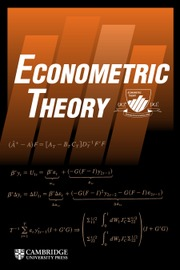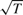Home
Hostname: page-component-55597f9d44-54jdg Total loading time: 1.013 Render date: 2022-08-08T19:19:36.437Z Has data issue: true Feature Flags: { "shouldUseShareProductTool": true, "shouldUseHypothesis": true, "isUnsiloEnabled": true, "useRatesEcommerce": false, "useNewApi": true } hasContentIssue trueEconometric Theory

# ESTIMATORS FOR PERSISTENT AND POSSIBLY NONSTATIONARY DATA WITH CLASSICAL PROPERTIES

Published online by Cambridge University Press:  27 April 2012

*
*Address correspondence to Serena Ng, Department of Economics, Columbia University, 420 W. 118 St., New York, NY 10027, USA; e-mail: Serena.Ng@columbia.edu.

## Abstract

This paper considers a moments-based nonlinear estimator that is$\root \of T$-consistent and uniformly asymptotically normal irrespective of the degree of persistence of the forcing process. These properties hold for linear autoregressive models, linear predictive regressions, and certain nonlinear dynamic models. Asymptotic normality is obtained because the moments are chosen so that the objective function is uniformly bounded in probability and so that a central limit theorem can be applied. Critical values from the normal distribution can be used irrespective of the treatment of the deterministic terms. Simulations show that the estimates are precise and the t-test has good size in the parameter region where the least squares estimates usually yield distorted inference.

Type
Articles
Information
Econometric Theory , October 2012 , pp. 1003 - 1036

## Access options

Get access to the full version of this content by using one of the access options below. (Log in options will check for institutional or personal access. Content may require purchase if you do not have access.)

## References

Abowd, J.M. & Card, D. (1989) On the covariance structure of earnings and hours changes. Econometrica 57, 411445.CrossRefGoogle Scholar
Altonji, J.G. & Segal, L.M. (1996) Small-sample bias in GMM estimation of covariance structures. Journal of Business & Economic Statistics 14, 353366.Google Scholar
Canjels, E. & Watson, M.W. (1997) Estimating deterministic trends in the presence of serially correlated errors. Review of Economics and Statistics 79, 184200.CrossRefGoogle Scholar
Chernozhukov, V. & Hong, H. (2003) An MCMC approach to classical estimation. Journal of Econometrics 115, 293346.CrossRefGoogle Scholar
Coibion, O. & Gorodnichenko, Y. (2011) Strategic interaction among heterogeneous price setters in estimated DSGE model. Review of Economics and Statistics 93, 920940.CrossRefGoogle Scholar
Gorodnichenko, Y. & Ng, S. (2010) Estimation of DSGE models when the data are persistent. Journal of Monetary Economics 57, 325340.CrossRefGoogle Scholar
Han, C. & Kim, B. (2011) A GMM interpretation of the paradox in the inverse probability weighting estimation of the average treatment effect on the treated. Economics Letters 110, 163165.CrossRefGoogle Scholar
Han, C., Phillips, P.C.B., & Sul, D. (2011) Uniform asymptotic normality in stationary and unit root autoregression. Econometric Theory 27, 11171151.CrossRefGoogle Scholar
Jansson, M. & Moreira, M.J. (2006) Optimal inference in regession models with nearly integrated regressors. Econometrica 74, 681714.CrossRefGoogle Scholar
Komunjer, I. & Ng, S. (2011) Dynamic identification of dynamic stochastic general equilibrium models. Econometrica 79, 19952032.Google Scholar
Laroque, G. & Salanie, B. (1997) Normal estimators for cointegrating relationships. Economics Letters 55, 185189.CrossRefGoogle Scholar
Mikusheva, A. (2007a) Uniform inference in autoregressive models. Econometrica 75, 14111452.CrossRefGoogle Scholar
Mikusheva, A. (2007b) Uniform inference in autoregressive models. Econometrica 75, online supplementary material.CrossRefGoogle Scholar
Mikusheva, A. (2012) One dimensional inference in autogressive models with potential presence of a unit root. Econometrica 80, 163212.Google Scholar
Newey, W.K. & McFadden, D. (1994) Large sample estimation and hypothesis testing. In Engle, R.F. & McFadden, D. (eds.), Handbook of Econometrics, vol. 4, pp. 21112245. North-Holland.Google Scholar
Pesavento, E. & Rossi, B. (2006) Small-sample confidence interevals for multivariate impulse response functions at long horizons. Journal of Applied Econometrics 21, 11351155.CrossRefGoogle Scholar
Phillips, P.C.B. & Han, C. (2008) Gaussian inference in AR(1) time series with or without a unit root. Econometric Theory 24, 631650.CrossRefGoogle Scholar
Phillips, P.C.B. & Lee, C.C. (1996) Efficiency gains from quasi-differencing under nonstationarity. In Robinson, P. & Rosenblatt, M. (eds.), Athens Conference on Applied Probability and Time Series, vol. II, Time Series Analysis in Honor of E.J. Hannan, pp. 300314. Springer.CrossRefGoogle Scholar
Phillips, P.C.B. & Solo, V. (1992) Asymptotics for linear processes. Annals of Statistics 20, 9711001.CrossRefGoogle Scholar
Phillips, P.C.B. & Xiao, Z. (1998) A primer on unit root testing. Journal of Economic Surveys 12, 423469.CrossRefGoogle Scholar
Pierce, D.A. (1982) The asymptotic effect of substituting estimators for parameters in certain types of statistics. Annals of Statistics 10, 475478.CrossRefGoogle Scholar
Prokhorov, A. & Schmidt, P. (2009) GMM redundancy results for general missing data problem. Journal of Econometrics 151, 4755.CrossRefGoogle Scholar
So, B.S. & Shin, D.W. (1999) Cauchy estimators for autoregressive processes with applications to unit root tests and confidence intervals. Econometric Theory 15, 165176.CrossRefGoogle Scholar
Uhlig, H. (1999) A Toolkit for analyzing nonlinear dynamic stochastic models easily. In Marimon, R. & Scott, A. (eds.), Computational Methods for the Study of Dynamic Economies, pp. 3061. Oxford University Press.Google Scholar
6
Cited by

# Save article to Kindle

Note you can select to save to either the @free.kindle.com or @kindle.com variations. ‘@free.kindle.com’ emails are free but can only be saved to your device when it is connected to wi-fi. ‘@kindle.com’ emails can be delivered even when you are not connected to wi-fi, but note that service fees apply.

Find out more about the Kindle Personal Document Service.

ESTIMATORS FOR PERSISTENT AND POSSIBLY NONSTATIONARY DATA WITH CLASSICAL PROPERTIES
Available formats
×

# Save article to Dropbox

To save this article to your Dropbox account, please select one or more formats and confirm that you agree to abide by our usage policies. If this is the first time you used this feature, you will be asked to authorise Cambridge Core to connect with your Dropbox account. Find out more about saving content to Dropbox.

ESTIMATORS FOR PERSISTENT AND POSSIBLY NONSTATIONARY DATA WITH CLASSICAL PROPERTIES
Available formats
×

# Save article to Google Drive

To save this article to your Google Drive account, please select one or more formats and confirm that you agree to abide by our usage policies. If this is the first time you used this feature, you will be asked to authorise Cambridge Core to connect with your Google Drive account. Find out more about saving content to Google Drive.

ESTIMATORS FOR PERSISTENT AND POSSIBLY NONSTATIONARY DATA WITH CLASSICAL PROPERTIES
Available formats
×
×